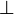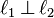# Right angle facts for kids

Kids Encyclopedia Facts
(Redirected from Right angles)

A right angle is an angle with a measurement of 90 degrees. When two lines cross each other so that all the angles have the same size, the result is four right angles. The top of the letter T is at right angles to the vertical line. Walls of buildings are usually at right angles to the floor. Two things that are at right angles are called "perpendicular" or "orthogonal". This is expressed using the$\bot$ symbol (such as in$\ell_1 \, \bot \, \ell_2$).

Planes (flat surfaces) can also meet at right angles. In a building, a wall and a floor are said to be perpendicular to each other, and they have a right angle. It also can be called a square angle.

## Images for kidsRight angle Facts for Kids. Kiddle Encyclopedia.